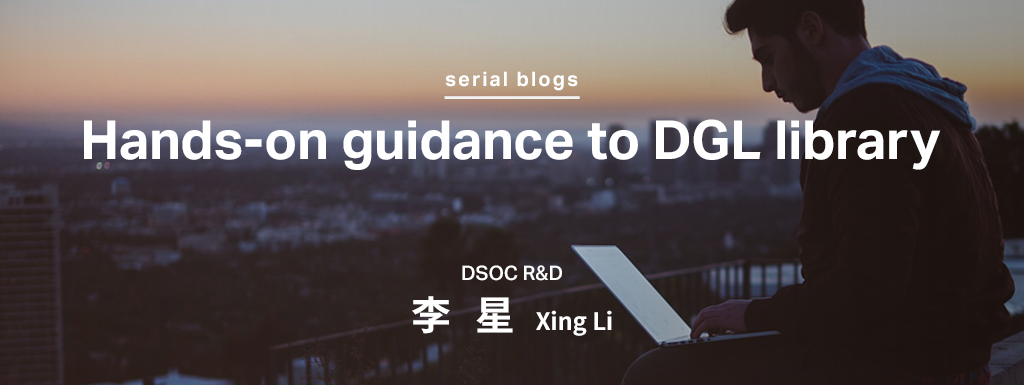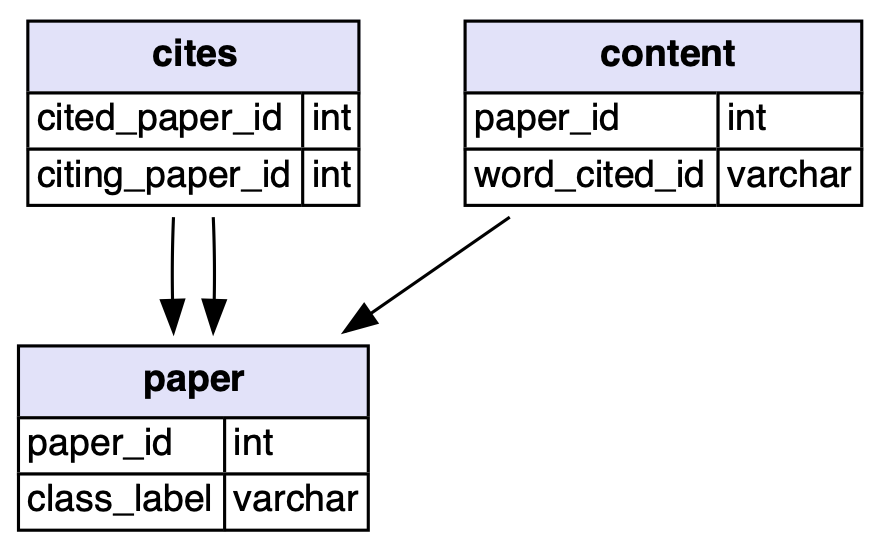# Hands-on guidance to DGL library _ (4) An introduction to training graph neural networksHi, I am XING LI, a researcher from Sansan DSOC.

We have discussed some of the most important elements in DGL, such as Message Passing and NodeFlow. And we also explored a small but vital topic in most deep neural networks: (Negative) Sampling Methods. These are most of the tools we need to master for building deep graph learning neural networks. Today, we are going to briefly introduce some common tasks in deep graph learning and build a toy network focused on one of these tasks as a demo.

# Common tasks in graph learning

One of the most popular and widely applied tasks for graph neural networks is Node Classification, where we need to predict nodes' true categories. If the task is supervised, we could observe some nodes are labelled as a ground truth category from a set of predefined categories. Conversely, the unsupervised setting will not provide any extra node category information.

Similarly, we may wish to predict the categories of edges, this is known as Edge Classification. One special sub-task of edge classification is called Link Prediction, under this setting we are going to predict whether an edge exists between two given nodes or not.

The above two tasks are all mainly based on "node information", "edge information" and "graph structure(connection) information" in one single graph to infer.

Instead of a single graph, sometimes we might have the data in the form of multiple graphs, for example, a list of different types of communities of people. In this scenario, a graph classification model could help identify the type of the community, i.e. to classify each graph based on the structure and overall information. Such a task is called a Graph Classification task.

# A concrete example of Node Classification task with help of DGL

The dataset used here is Cora dataset, which is the MNIST equivalent in graph learning. The Cora dataset consists of 2708 scientific publications classified into one of seven classes. The citation network consists of 5429 links. Each publication in the dataset is described by a 0/1-valued word vector indicating the absence/presence of the corresponding word from the dictionary. The dictionary consists of 1433 unique words. Hence it is a directional homogeneous graph. The data diagram and visualisation are as follows:The data diagram of Cora dataset

Load some necessary packages first:

import torch
import torch as th
import torch.nn as nn
import torch.nn.functional as F

import dgl
from dgl import DGLGraph
from dgl.data import CoraGraphDataset
import dgl.function as fn

import time
import networkx as nx
import numpy as np
import matplotlib.pyplot as plt
%config InlineBackend.figure_format = 'svg'


We can load Cora dataset through DGL’s built-in data module:

dataset = CoraGraphDataset()
graph = dataset


It shows information like:

'''
Output:
NumNodes: 2708
NumEdges: 10556
NumFeats: 1433
NumClasses: 7
NumTrainingSamples: 140
NumValidationSamples: 500
NumTestSamples: 1000
Done saving data into cached files.
'''


We can see the graph's preprocessed information:

graph.ndata

'''
Output:
{'train_mask': tensor([ True,  True,  True,  ..., False, False, False]), 'val_mask': tensor([False, False, False,  ..., False, False, False]), 'test_mask': tensor([False, False, False,  ...,  True,  True,  True]), 'label': tensor([3, 4, 4,  ..., 3, 3, 3]), 'feat': tensor([[0., 0., 0.,  ..., 0., 0., 0.],
[0., 0., 0.,  ..., 0., 0., 0.],
[0., 0., 0.,  ..., 0., 0., 0.],
...,
[0., 0., 0.,  ..., 0., 0., 0.],
[0., 0., 0.,  ..., 0., 0., 0.],
[0., 0., 0.,  ..., 0., 0., 0.]])}
'''


Cora naturally contains seven classes, and statistics below show that each class does satisfy our assumption of community, i.e. nodes of the same class have higher connection probability among them than with nodes of a different class. The following code snippet verifies that there are more intra-class edges than inter-class (>0.5).

# find all the nodes labelled with class 0
label0_nodes = th.nonzero(graph.ndata['label'] == 0).squeeze()

# find all the edges pointing to class 0 nodes
src, _ = graph.in_edges(label0_nodes)
src_labels = graph.ndata['label'][src]

# find all the edges whose both endpoints are in class 0
intra_src = th.nonzero(src_labels == 0)
print('Intra-class edges percent: %.4f' % (len(intra_src) / len(src_labels)))

'''
Output:
Intra-class edges percent: 0.6994
'''


We first define the message and reduce function as we discussed in series (1) by built-in functions.

gcn_msg = fn.copy_src(src='h', out='m')
gcn_reduce = fn.sum(msg='m', out='h')


We then proceed to define the GCNLayer module . A GCNLayer essentially performs message passing on all the nodes then applies a fully-connected layer.

class GCNLayer(nn.Module):
def __init__(self, in_feats, out_feats):
super(GCNLayer, self).__init__()
self.linear = nn.Linear(in_feats, out_feats)

def forward(self, g, feature):
with g.local_scope():
g.ndata['h'] = feature
g.update_all(gcn_msg, gcn_reduce)
h = g.ndata['h']
return self.linear(h)


Let’s define a simple neural network consisting of two GCN layers. Suppose we are training the classifier for the Cora dataset (the input feature size is 1433 and the number of classes is 7):

class Net(nn.Module):
def __init__(self):
super(Net, self).__init__()
self.layer1 = GCNLayer(1433, 16)
self.layer2 = GCNLayer(16, 7)

def forward(self, g, features):
x = F.relu(self.layer1(g, features))
x = self.layer2(g, x)
return x


Then wen can use the following method to evaluate the performance of the model on the test dataset:

def evaluate(model, g, features, labels, mask):
model.eval()
logits = model(g, features)
_, indices = th.max(logits, dim=1)
correct = th.sum(indices == labels)
return correct.item() * 1.0 / len(labels)


Initialise the network and train it:

net = Net()
print(net)

'''
Output:
Net(
(layer1): GCNLayer(
(linear): Linear(in_features=1433, out_features=16, bias=True)
)
(layer2): GCNLayer(
(linear): Linear(in_features=16, out_features=7, bias=True)
)
)
'''

optimizer = th.optim.Adam(net.parameters(), lr=1e-3)
dur = []
for epoch in range(250):
t0 = time.time()

net.train()
logits = net(graph, graph.ndata['feat'])
logp = F.log_softmax(logits, 1)

loss.backward()
optimizer.step()

acc = evaluate(net, graph, graph.ndata['feat'], graph.ndata['label'], graph.ndata['test_mask'])
if epoch%10==0:
print("Epoch {:05d} | Loss {:.4f} | Test Acc {:.4f} | Time(s) {:.4f}".format(
epoch, loss.item(), acc, time.time()-t0))
'''
Output:
Epoch 00000 | Loss 1.9636 | Test Acc 0.1300 | Time(s) 0.0235
Epoch 00010 | Loss 1.7854 | Test Acc 0.3050 | Time(s) 0.0216
Epoch 00020 | Loss 1.6293 | Test Acc 0.3950 | Time(s) 0.0214
Epoch 00030 | Loss 1.4923 | Test Acc 0.4900 | Time(s) 0.0206
Epoch 00040 | Loss 1.3688 | Test Acc 0.5870 | Time(s) 0.0213
Epoch 00050 | Loss 1.2568 | Test Acc 0.6440 | Time(s) 0.0206
Epoch 00060 | Loss 1.1539 | Test Acc 0.6760 | Time(s) 0.0215
Epoch 00070 | Loss 1.0584 | Test Acc 0.7010 | Time(s) 0.0212
Epoch 00080 | Loss 0.9702 | Test Acc 0.7080 | Time(s) 0.0205
Epoch 00090 | Loss 0.8892 | Test Acc 0.7100 | Time(s) 0.0200
Epoch 00100 | Loss 0.8156 | Test Acc 0.7180 | Time(s) 0.0200
Epoch 00110 | Loss 0.7492 | Test Acc 0.7200 | Time(s) 0.0205
Epoch 00120 | Loss 0.6895 | Test Acc 0.7220 | Time(s) 0.0201
Epoch 00130 | Loss 0.6358 | Test Acc 0.7190 | Time(s) 0.0210
Epoch 00140 | Loss 0.5875 | Test Acc 0.7190 | Time(s) 0.0214
Epoch 00150 | Loss 0.5440 | Test Acc 0.7300 | Time(s) 0.0199
Epoch 00160 | Loss 0.5046 | Test Acc 0.7320 | Time(s) 0.0205
Epoch 00170 | Loss 0.4690 | Test Acc 0.7340 | Time(s) 0.0216
Epoch 00180 | Loss 0.4368 | Test Acc 0.7330 | Time(s) 0.0215
Epoch 00190 | Loss 0.4074 | Test Acc 0.7330 | Time(s) 0.0216
Epoch 00200 | Loss 0.3807 | Test Acc 0.7290 | Time(s) 0.0215
Epoch 00210 | Loss 0.3564 | Test Acc 0.7290 | Time(s) 0.0202
Epoch 00220 | Loss 0.3341 | Test Acc 0.7280 | Time(s) 0.0216
Epoch 00230 | Loss 0.3136 | Test Acc 0.7280 | Time(s) 0.0205
Epoch 00240 | Loss 0.2948 | Test Acc 0.7270 | Time(s) 0.0209
'''


That is it! We easily achieve 0.72 accuracy score by running the above simple codes in Cora dataset node classification task!

Now you have known how to build, train and test a simple deep graph neural network by DGL. In today's case, the graph only have 2708 nodes, the aggregation operation will not take a too long time. However, we may face much larger graphs, for example over million nodes and billion edges, in the real world. Also, the NN architecture would be more complex, the computation requirements would be heavier, then we have to consider the scalability problem of training and inference of such huge deep graph neural networks. We will discuss these contents in the next blog. See you~

# References

 Kipf, T.N. and Welling, M., 2016. Semi-supervised classification with graph convolutional networks. arXiv preprint arXiv:1609.02907.